### Mixture Models Contrasted with Mixing and Mixed Models: Untangling the Terminology Web

Statistical terminology can have its limitations. The terms mixture, mixing, and mixed models are sometimes used interchangeably, causing confusion. Even worse, the terms arise in related situations. One application needs to be eliminated from the discussion in this documentation: mixture experiments, where design factors are the proportions with which components contribute to a blend, are not mixture models and do not fall under the purview of the FMM procedure. However, the data from a mixture experiment might be analyzed with a mixture model, a mixing model, or a mixed model, besides other types of statistical models.

Suppose that you observe realizations of random variable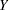and assume thatfollows some distribution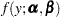that depends on parameters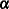and. Furthermore, suppose that the model is found to be deficient in the sense that the variability implied by the fitted model is less than the observed variability in the data, a condition known as overdispersion (see the section Overdispersion). To tackle the problem the statistical model needs to be modified to allow for more variability. Clearly, one way of doing this is to introduce additional random variables into the process. Mixture, mixing, and mixed models are simply different ways of adding such random variables. The section The Form of the Finite Mixture Model explains how mixture models add a discrete state variable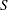. The following two subsections explain how mixing and mixed models instead assume variation for a natural parameter or in the mean function.

#### Mixing Models

Suppose that the model is modified to allow for some random quantity
, which might be one of the parameters of the model or a quantity related to the parameters. Now there are two distributions to cope with: the conditional distribution of the response given the random effect,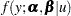and the marginal distribution of the data. Ifis continuous, the marginal distribution is obtained by integration: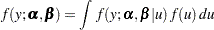Otherwise, it is obtained by summation over the support of: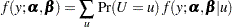The important entity for statistical estimation is the marginal distribution; the conditional distribution is often important for model description, genesis, and interpretation.

In a mixing model the marginal distribution is known and is typically of a well-known form. For example, if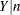has a binomial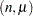distribution andfollows a Poisson distribution, then the marginal distribution ofis Poisson. The preceding operation is called mixing a binomial distribution with a Poisson distribution. Similarly, when mixing a Poisson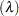distribution with a gamma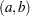distribution for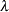, a negative binomial distribution results as the marginal distribution. Other important mixing models involve mixing a binomialrandom variable with a betadistribution for the binomial success probability. This results in a distribution known as the beta-binomial.

The finite mixtures have in common with the mixing models the introduction of random effects into the model to vary some or all of the parameters at random.

#### Mixed Models

The difference between a mixing and a mixed model is that the conditional distribution is not that important in the mixing model. It matters to motivate the overdispersed reference model and to arrive at the marginal distribution. Inferences with respect to the conditional distribution, such as predicting the random variable, are not performed in mixing models. In a mixed model the random variabletypically follows a continuous distribution—almost always a normal distribution. The random effects usually do not model the natural parameters of the distribution; instead, they are involved in linear predictors that relate to the conditional mean. For example, a linear mixed model is a model in which the response and the random effects are normally distributed, and the random effects enter the conditional mean function linearly: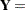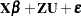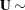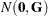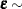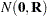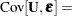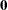The conditional and marginal distributions are then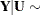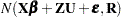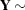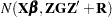For this model, because of the linearity in the mean and the normality of the random effects, you could also refer to mixing the normal vector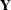with the normal vector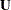, since the marginal distribution is known. The linear mixed model can be fit with the MIXED procedure. When the conditional distribution is not normal and the random effects are normal, the marginal distribution does not have a closed form. In this class of mixed models, called generalized linear mixed models, model approximations and numerical integration methods are commonly used in model fitting; see for example, those models fit by the GLIMMIX and NLMIXED procedures. Chapter 6, Introduction to Mixed Modeling Procedures, contains details about the various classes of mixed models and about the relevant SAS/STAT procedures.

The previous expression for the marginal variance in the linear mixed model,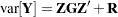, emphasizes again that the variability in the marginal distribution of a model that contains random effects exceeds the variability in a model without the random effects (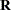).

The finite mixtures have in common with the mixed models that the marginal distribution is not necessarily a well-known model, but is expressed through a formal integration over the random-effects distribution. In contrast to the mixed models, in particular those involving nonnormal distributions or nonlinear elements, this integration is rather trivial; it reduces to a weighted and finite sum of densities or mass functions.